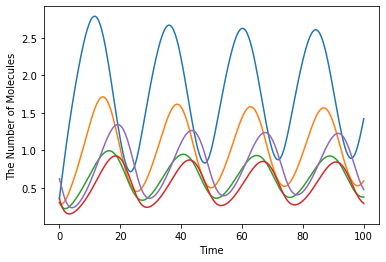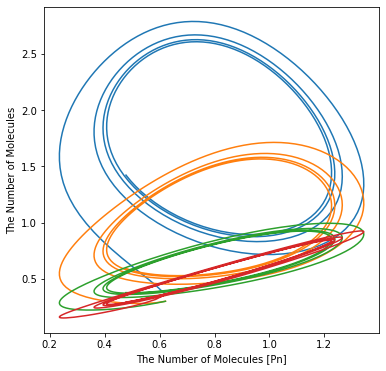:

%matplotlib inline
from ecell4.prelude import *


This is a model of the oscillating Drosophila period protein (PER). This model is based on the model introduced in the following publication:

:

citation(8587874)


Goldbeter A, A model for circadian oscillations in the Drosophila period protein (PER). Proceedings. Biological sciences, 1362(261), 319-24, 1995. 10.1098/rspb.1995.0153. PubMed PMID: 8587874.

:

with reaction_rules():
~M > M | 0.76 / (1 + Pn ** 3)
M > ~M | 0.65 * M / (0.5 + M)
~P0 > P0 | 0.38 * M
P0 == P1 | (3.2 * P0 / (2 + P0), 1.58 * P1 / (2 + P1))
P1 == P2 | (5 * P1 / (2 + P1), 2.5 * P2 / (2 + P2))
P2 == Pn | (1.9, 1.3)
P2 > ~P2 | 0.95 * P2 / (0.2 + P2)

m = get_model()

:

y0 = {"M": 3.61328202e-01, "Pn": 6.21367e-01, "P0": 3.01106835e-01, "P1": 3.01106835e-01, "P2": 3.61328202e-01}
ret = run_simulation(100, ndiv=400, model=m, y0=y0)

:

ret:

ret.plot(x="Pn", y=["M", "P0", "P1", "P2"], figsize=[6.0, 6.0])